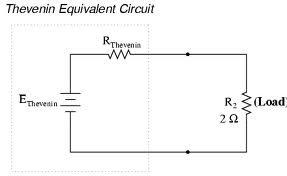## Thevenins Equivalent Circuit Assignment Help

Assignment Help: >> AC Circuits - Thevenins Equivalent Circuit

Thevenin's Equivalent Circuit:Figure: Thevenin's Equivalent Circuit

Where VTH = Thevenin's equivalent voltage

= VOC = Open circuit voltage across the load terminals.

ZTH = Thevenin's equivalent impedance

= Drive point impedance across the load terminals when all of the sources replaced by their internal resistances.